Test: Trigonometric Functions- Case Based Type Questions

# Test: Trigonometric Functions- Case Based Type Questions

Test Description

## 10 Questions MCQ Test Mathematics (Maths) Class 11 | Test: Trigonometric Functions- Case Based Type Questions

Test: Trigonometric Functions- Case Based Type Questions for Commerce 2023 is part of Mathematics (Maths) Class 11 preparation. The Test: Trigonometric Functions- Case Based Type Questions questions and answers have been prepared according to the Commerce exam syllabus.The Test: Trigonometric Functions- Case Based Type Questions MCQs are made for Commerce 2023 Exam. Find important definitions, questions, notes, meanings, examples, exercises, MCQs and online tests for Test: Trigonometric Functions- Case Based Type Questions below.
Solutions of Test: Trigonometric Functions- Case Based Type Questions questions in English are available as part of our Mathematics (Maths) Class 11 for Commerce & Test: Trigonometric Functions- Case Based Type Questions solutions in Hindi for Mathematics (Maths) Class 11 course. Download more important topics, notes, lectures and mock test series for Commerce Exam by signing up for free. Attempt Test: Trigonometric Functions- Case Based Type Questions | 10 questions in 20 minutes | Mock test for Commerce preparation | Free important questions MCQ to study Mathematics (Maths) Class 11 for Commerce Exam | Download free PDF with solutions
 1 Crore+ students have signed up on EduRev. Have you?
Test: Trigonometric Functions- Case Based Type Questions - Question 1

### A quadrilateral ABCD is inscribed in a circle as shown in the figure.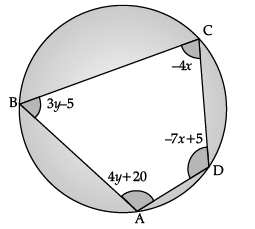Based on the above data, answer the following question.Q. The value of y is .............

Detailed Solution for Test: Trigonometric Functions- Case Based Type Questions - Question 1 y = x + 40° = -15° + 40° = 25°
Test: Trigonometric Functions- Case Based Type Questions - Question 2

### A quadrilateral ABCD is inscribed in a circle as shown in the figure.Based on the above data, answer the following question.Q. cos A + cos B + cos C + cos D = ...........

Detailed Solution for Test: Trigonometric Functions- Case Based Type Questions - Question 2 cos A + cos B + cos C + cos D

= cos A + cos B + cos(180° – A) + cos(180° – B)

= cos A + cos B – cos A – cos B = 0

Test: Trigonometric Functions- Case Based Type Questions - Question 3

### A quadrilateral ABCD is inscribed in a circle as shown in the figure.Based on the above data, answer the following question.Q. cos(A + B + C + D) = .............

Detailed Solution for Test: Trigonometric Functions- Case Based Type Questions - Question 3 Since ABCD is a quadrilateral,

A + B + C + D = 360°

cos(A + B + C + D) = cos 360° = 1

Test: Trigonometric Functions- Case Based Type Questions - Question 4

A quadrilateral ABCD is inscribed in a circle as shown in the figure.Based on the above data, answer the following question.

Q. sin (B + D) = ..............

Detailed Solution for Test: Trigonometric Functions- Case Based Type Questions - Question 4 Since ABCD is a cyclic quadrilateral,

B + D = 180° (opposite angles are supplementary)

∴ sin(B + D) = sin 180° = 0

Test: Trigonometric Functions- Case Based Type Questions - Question 5

A quadrilateral ABCD is inscribed in a circle as shown in the figure.Based on the above data, answer the following question.

Q. The value of x is ..............

Detailed Solution for Test: Trigonometric Functions- Case Based Type Questions - Question 5 A + C = 180°

⇒ - 4x + 4y + 20 = 180°

- 4x + 4y = 160°

x - y = - 40° ...(i)

B + D = 180°

⇒ 3y - 5 - 7x + 5 = 180°

- 7x + 3y = 180° ...(ii)

Solving (i) and (ii), x = –15°

Test: Trigonometric Functions- Case Based Type Questions - Question 6

A compound angle is an angle which can be expressed as the sum or difference of two standard angles.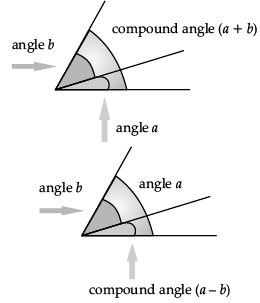Based on the above data, answer the following question.

Q. The value of tan 75° is = .............

Detailed Solution for Test: Trigonometric Functions- Case Based Type Questions - Question 6 tan 75° = tan(45° + 30°)

=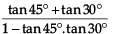=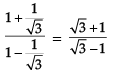= 2 + √3 (after rationalising denominator)

Test: Trigonometric Functions- Case Based Type Questions - Question 7

A compound angle is an angle which can be expressed as the sum or difference of two standard angles.Based on the above data, answer the following question.

Q. sin(A + B).sin(A - B) = .............

Detailed Solution for Test: Trigonometric Functions- Case Based Type Questions - Question 7 sin(A + B).sin(A – B)

= (sin A. cos B + cos A. sin B)(sin A. cos B - cos A. sin B)

= (sin A. cos B)2 - (cos A. sin B)2

= sin2 A. cos2 B - cos2 A. sin2 B

= sin2 A (1 - sin2 B) - (1 - sin2 A) sin2 B

= sin2 A - sin2 A. sin2 B - sin2 B + sin2 A. sin2 B

= sin2 A - sin2 B

Test: Trigonometric Functions- Case Based Type Questions - Question 8

A compound angle is an angle which can be expressed as the sum or difference of two standard angles.Based on the above data, answer the following question.

Q. The value of cos 75° is ..............

Detailed Solution for Test: Trigonometric Functions- Case Based Type Questions - Question 8 cos 75° = cos(30° + 45°)

= cos 30°.cos 45° – sin 30°.sin 45°

=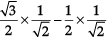=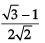Test: Trigonometric Functions- Case Based Type Questions - Question 9

A compound angle is an angle which can be expressed as the sum or difference of two standard angles.Based on the above data, answer the following question.

Q. 1 + cos 2A = ............

Detailed Solution for Test: Trigonometric Functions- Case Based Type Questions - Question 9 cos 2 A = cos (A + A)

= cos A. cos A – sin A. sin A

= cos2 A – sin2 A

= cos2 A – (1 – cos2 A)

= 2 cos2 A – 1

∴ 1 + cos 2 A = 2 cos2 A

Test: Trigonometric Functions- Case Based Type Questions - Question 10

A compound angle is an angle which can be expressed as the sum or difference of two standard angles.Based on the above data, answer the following question.

Q. The value of sin 75° is ..............

Detailed Solution for Test: Trigonometric Functions- Case Based Type Questions - Question 10 sin 75° = sin(30° + 45°)

= sin 30°.cos 45° + cos 30°.sin 45°

=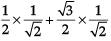=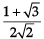## Mathematics (Maths) Class 11

156 videos|176 docs|132 tests
 Use Code STAYHOME200 and get INR 200 additional OFF Use Coupon Code
Information about Test: Trigonometric Functions- Case Based Type Questions Page
In this test you can find the Exam questions for Test: Trigonometric Functions- Case Based Type Questions solved & explained in the simplest way possible. Besides giving Questions and answers for Test: Trigonometric Functions- Case Based Type Questions, EduRev gives you an ample number of Online tests for practice

## Mathematics (Maths) Class 11

156 videos|176 docs|132 tests